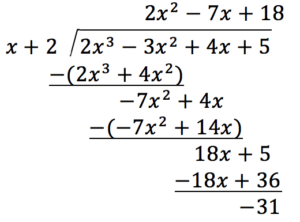## Synthetic Division

### Learning Outcomes

• Use synthetic division to divide polynomials.

As we’ve seen, long division with polynomials can involve many steps and be quite cumbersome. Synthetic division is a shorthand method of dividing polynomials for the special case of dividing by a linear factor whose leading coefficient is 1.

To illustrate the process, recall the example at the beginning of the section.

Divide $2{x}^{3}-3{x}^{2}+4x+5$ by $x+2$ using the long division algorithm.

The final form of the process looked like this:There is a lot of repetition in the table. If we don’t write the variables but instead line up their coefficients in columns under the division sign and also eliminate the partial products, we already have a simpler version of the entire problem.

Synthetic division carries this simplification even a few more steps. Collapse the table by moving each of the rows up to fill any vacant spots. Also, instead of dividing by 2, as we would in division of whole numbers, and then multiplying and subtracting the middle product, we change the sign of the “divisor” to –2, multiply, and add. The process starts by bringing down the leading coefficient.

We then multiply it by the “divisor” and add, repeating this process column by column until there are no entries left. The bottom row represents the coefficients of the quotient; the last entry of the bottom row is the remainder. In this case, the quotient is $2x{^2} -7x+18$ and the remainder is –31. The process will be made more clear in the examples that follow.

### A General Note: Synthetic Division

Synthetic division is a shortcut that can be used when the divisor is a binomial in the form x – k. In synthetic division, only the coefficients are used in the division process.

### How To: Given two polynomials, use synthetic division to divide

1. Write k for the divisor.
2. Write the coefficients of the dividend.
3. Bring the leading coefficient down.
4. Multiply the leading coefficient by k. Write the product in the next column.
5. Add the terms of the second column.
6. Multiply the result by k. Write the product in the next column.
7. Repeat steps 5 and 6 for the remaining columns.
8. Use the bottom numbers to write the quotient. The number in the last column is the remainder and has degree 0, the next number from the right has degree 1, the next number from the right has degree 2, and so on.

### Example: Using Synthetic Division to Divide a Second-Degree Polynomial

Use synthetic division to divide $5{x}^{2}-3x - 36$ by $x - 3$.

### Example: Using Synthetic Division to Divide a Third-Degree Polynomial

Use synthetic division to divide $4{x}^{3}+10{x}^{2}-6x - 20$ by $x+2$.

### Example: Using Synthetic Division to Divide a Fourth-Degree Polynomial

Use synthetic division to divide $-9{x}^{4}+10{x}^{3}+7{x}^{2}-6$ by $x - 1$.

### Try It

Use synthetic division to divide $3{x}^{4}+18{x}^{3}-3x+40$ by $x+7$.

https://www.myopenmath.com/multiembedq.php?id=29483&theme=oea&iframe_resize_id=mom1

Polynomial division can be used to solve a variety of application problems involving expressions for area and volume. We looked at an application at the beginning of this section. Now we will solve that problem in the following example.

### Example: Using Polynomial Division in an Application Problem

The volume of a rectangular solid is given by the polynomial $3{x}^{4}-3{x}^{3}-33{x}^{2}+54x$. The length of the solid is given by 3x and the width is given by x – 2. Find the height of the solid.

### Try It

The area of a rectangle is given by $3{x}^{3}+14{x}^{2}-23x+6$. The width of the rectangle is given by + 6. Find an expression for the length of the rectangle.

## Contribute!

Did you have an idea for improving this content? We’d love your input.